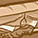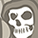Attachments are accessible only for community members.
Log in# Distance2Go Evaluate recorded data from RadarGUILevel 3
Level 3Hello,

I'm trying to evaluate data I recorded with the RadarGUI to get the same results as the RadarGUI shows me. I'm working with a Distance2Go Demo Board.
The D2G board detects two targets and displays both in the RadarGUI, so far so good. Then I used the record function to record the IQ data, the FFT data and the target info data.

Now I'm trying to evaluate the recorded FFT data to get the same target info (range and level) as the RadarGUI shows me. However, I cannot get it to work (I'm using MATLAB).
The recored FFT data (can be easily pasted into MATLAB) is attached to this post. The FFT bin size is the sampling frequency divided by the FFT size which is 166666 Hz/1024=162.76 Hz. To get the desired info I should have peaks with a beatfrequency as follows:
1304.6 Hz, 2609.3 Hz. Therefore the results I should get are: target 1) level: 1341, range 260 cm and target 2) level: 882, range 139 cm. So the 1304 Hz are connected to target #2, and the 2609 Hz to target #1.

I am trying to find the FFT peaks with the following MATLAB function:
`[lvl,idx]=findpeaks(fftdata,'MinPeakHeight',10); `
, where 10 is the range threshold and fftdata is a vector that contains the data from the attached file.
The values in lvl correspond to the levels mentioned above. But there is a problem with the beatfrequency. I calculate it by
`fbeat=idx.*binsize;`
. By doing so, I don't end up with the frequencies mentioned above. What am I doing wrong?

Another problem is that I get 11 instead of 2 results by doing that...

4 Replies

# Re: Distance2Go Evaluate recorded data from RadarGUIEmployee
Employee
Hi,

The Sampling Frequency that you are talking about is indeed the ADC sampling rate (Number of samples per second). The ADC sampling rate is given by: (4*Bandwidth*Max_distance)/(c_light*T_chirp).
Secondly, the value '10' used as Threshold to find peaks is very low. This is reason you observe 11 peaks instead of 2.
You can use the following method to compute the target ranges from the FFT data.

threshold=300;% Range Threshold
[lvl,idx]=findpeaks(fftdata,'MinPeakHeight',threshold);
c0=3e8; % Speed of light in vacuum
BW=250e6; % Bandwidth
NTS=250; % Number of ADC samples per chirp
T_chirp=1.5e-3; % Up chirp duration
RANGE_FFT_SIZE=1024;
R_max = NTS*c0/(2*BW); % Maximum Range attainable by the system
DistPerBin = R_max/(RANGE_FFT_SIZE); %Distance Per frequency bin
dist_vec = linspace(0,(R_max-R_max/(RANGE_FFT_SIZE))/2, RANGE_FFT_SIZE/2); % distance vector in m
Range=(idx-1) * DistPerBin; % Target ranges
Target_strength=lvl;
f_b=(2*BW*Range)./(c0*T_chirp); % Target beat frequency
% Plot the fftdata
figure();
plot(dist_vec,A);
xlabel('Range (m)');
ylabel('FFT')

This should give you the values of the target ranges and corresponding beat frequencies.
Hopefully this helps you.

# Re: Distance2Go Evaluate recorded data from RadarGUILevel 3
Level 3Hello Vaishnav,

Just one question: What is 'A' supposed to be? You are using it for plotting but it is not defined anywhere before?
I'll try out your example later and give you response. Maybe I'll figure out what A is by myself.

Thanks!

# Re: Distance2Go Evaluate recorded data from RadarGUILevel 3
Level 3Hey,
sorry for the late respone, but it is working fine. I'm using the fftdata as A.
I understand that the threshold 10 might be a bit too low but I'm still having a problem that when I set the threshold value to the same value as in the Radar GUI I keep getting different results. But it is okay as it is now, I can work with that!

# Re: Distance2Go Evaluate recorded data from RadarGUILevel 3
Level 3I just stumbled across another question...:

Vaishnav wrote:

The Sampling Frequency that you are talking about is indeed the ADC sampling rate (Number of samples per second). The ADC sampling rate is given by: (4*Bandwidth*Max_distance)/(c_light*T_chirp).

If I rearrange the formula it is (calling Max_distance=R_max):
R_max = (f_Sampling*c*T_chirp)/(4*Bandwidth).
With f_Sampling=167 kHz (as mentioned above), c=3e8 m/s, T_chirp=1500 us, Bandwidth=250 MHz I'm getting: 75 m for R_max